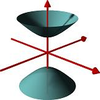#### You may also like### A Close Match

Can you massage the parameters of these curves to make them match as closely as possible?### Prime Counter

A short challenge concerning prime numbers.### The Right Volume

Can you rotate a curve to make a volume of 1?

##### Age 16 to 18 ShortChallenge Level

A mad robot sets off towards the North East on a journey from the origin. It travels in stages by moving forward and then rotating on the spot. It follows these pseudo-code instructions:

SUB JOURNEY

$\quad$DISTANCE = 1000

$\quad$WHILE (DISTANCE  > 0.001)
$\quad\quad$MOVE DISTANCE
$\quad\quad$STOP
$\quad\quad$ROTATE(90, DEGREES, CLOCKWISE)
$\quad\quad$DISTANCE = DISTANCE / 2
$\quad$END WHILE

$\quad$EXPLODE

END SUB

Where does the robot explode?

Extension: Write a program such that the angle rotated and other factors in the problem are variables. Explore where the robot might end up.

Did you know ... ?

Many computer programming jobs involve a mixture of logic, clear thinking and mathematics. Learning lots of mathematics and learning to think programmatically can open many doors in the job-market.